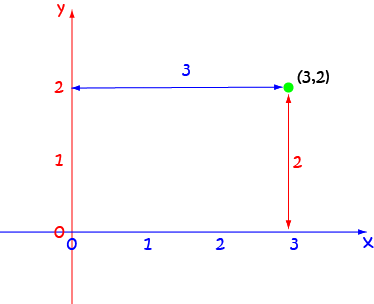# Definition of CoordinatesCoordinates tell us where a certain point is in the plane. They are represented by ordered pairs.

The coordinates of points in the $xy$-plane are often called Cartesian coordinates. The first element of each ordered pair tells us how far to the left or right the point is. The second element tells us how far up or down it is.

For example, the Cartesian coordinates $(3,2)$ tell us that the point is $3$ units to the right and $2$ units up.

If we are working in higher dimensional spaces, we need more coordinates. For example, in three-dimensions, we need three coordinates. In four dimensions, we need $4$ coordinates to exactly locate a point.

### Description

The aim of this dictionary is to provide definitions to common mathematical terms. Students learn a new math skill every week at school, sometimes just before they start a new skill, if they want to look at what a specific term means, this is where this dictionary will become handy and a go-to guide for a student.

### Audience

Year 1 to Year 12 students

### Learning Objectives

Learn common math terms starting with letter C

Author: Subject Coach
You must be logged in as Student to ask a Question.